# Stochastic Oscillator, the beginning - page 2587

Requested: Stochastic slope indicator

Can someone code this?20

Help Me!!!!

Hi guys..

i newbie in forex trading, i need your help to build a simple EA for me.

Can someone help me? i really appreciate if someone can help me.

my setting simple EA:-

TF M5

Stochastic (5.3.3)

TP 10PIPS

LOTS 0.1

enter position buy when line stoch cross at level 80

enter positon sell when line stoch cross at level 20

*sorry for my english

thanks.15

newdigital:
I tryed to collect everything about one indicator: Stochastic Oscillator.

"Technical Analysis from A to Z":

Overview

Sto.chas.tic (sto kas'tik) adj. 2. Math. designating a process

having an infinite progression of jointly

distributed random variables.

--- Webster's New World Dictionary

The Stochastic Oscillator compares where

a security's price closed relative to its

price range over a given time period.[/CODE]

Interpretation

The Stochastic Oscillator is displayed

as two lines. The main line is called "%K."

The second line, called "%D," is a

moving average of %K. The %K line is

usually displayed as a solid line and

the %D line is usually displayed as

a dotted line.

There are several ways to interpret

a Stochastic Oscillator. Three popular methods include:

Buy when the Oscillator (either %K or %D)

falls below a specific level (e.g., 20) and

then rises above that level. Sell when

the Oscillator rises above a specific level

(e.g., 80) and then falls below that level.

Buy when the %K line rises above

the %D line and sell when the %K line

falls below the %D line.

Look for divergences. For example,

where prices are making a series of new highs

and the Stochastic Oscillator is failing to surpass

its previous highs.

[CODE]Calculation

The Stochastic Oscillator has four variables:

%K Periods.

This is the number of time periods

used in the stochastic calculation.

%K Slowing Periods.

This value controls the internal smoothing of %K.

A value of 1 is considered a fast stochastic;

a value of 3 is considered a slow stochastic.

%D Periods.

This is the number of time periods used

when calculating a moving average of %K.

The moving average is called "%D" and is

usually displayed as a dotted line on

top of %K.

%D Method.

The method (i.e., Exponential,

Simple, Time Series, Triangular, Variable,

or Weighted) that is used to calculate %D.

The formula for %K is: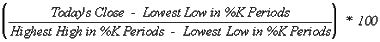For example, to calculate a 10-day %K,

first find the security's highest-high and

lowest-low over the last 10 days.

As an example, let's assume that during

the last 10 days the highest-high was 46 and

the lowest-low was 38--a range of 8 points.

If today's closing price was 41,

%K would be calculated as: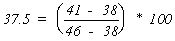The 37.5% in this example shows

that today's close was at the level of 37.5%

relative to the security's trading range over

the last 10 days. If today's close was 42,

the Stochastic Oscillator would be 50%.

This would mean that that the security

closed today at 50%, or the mid-point,

The above example used a

%K Slowing Period of 1-day (no slowing).

If you use a value greater than one,

you average the highest-high and

the lowest-low over the number of

%K Slowing Periods before performing

the division.

A moving average of %K is then

calculated using the number of time

periods specified in the %D Periods.

This moving average is called %D.

The Stochastic Oscillator always ranges between 0% and 100%. A reading of 0% shows that the security's close was the lowest price that the security has traded during the preceding x-time periods. A reading of 100% shows that the security's close was the highest price that the security has traded during the preceding x-time periods.

Do you have a study of indicator coded to calculate price where two K% (or D%) of two Stochastics crossover, and also to calculate price where K% cross the overbought/oversold level of the stochastic?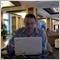Moderator
122849

khusha7765:
Do you have a study of indicator coded to calculate price where two K% (or D%) of two Stochastics crossover, and also to calculate price where K% cross the overbought/oversold level of the stochastic?

No. Sometimes I am using 3 stochastic indicators in 3 sepaated window with different settings for M5 timeframe. I did not try to combine those indicators onto one indicator. Simple did not need it.3203

stoch channel(Levels) on the chart [cw]

it's all wrong, but it works somehow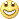stoh hooked on bbands istead of deviation

________________

double Stoch_Main1[];

Stoch_Main1 =iStochastic(NULL,0,14,3,3,MODE_SMA,0,MODE_MAIN, i);

double Stoch_Main =iStochastic(NULL,0,14,3,3,MODE_SMA,0,MODE_MAIN, i);

double Stoch_SigL=(iStochastic(NULL,0,14,3,3,MODE_SMA,0,MODE_SIGNAL, i));

double StochMain1=Stoch_Main1;

double StochMain=Stoch_Main;

double StochSigL=Stoch_SigL;

UpperBuffer=oldval +0.3*oldval*((100-StochMain1)/10000);

LowerBuffer=oldval - 0.3*oldval*((100-StochMain1)/10000);

UpperBuffer1=oldval + oldval*((StochMain-50)/10000);

LowerBuffer1=oldval + oldval*((StochSigL-50)/10000);

Files:
stoh_lvls_3.gif  25 kb3203

could some kind sole programmer find sanity in this medness & put it right?

thank you very much!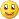3203

Big Thanks to Mladen - here is the indi - made nice and clean...(MTF option built-in) RSI of MA thread post181: https://www.mql5.com/en/forum/177347/page123203

OnChart_Stochastic_Channel_(FL_MTF)

from Mladen: Fibo Levels; MultiTimeFrameFiles:298

^^ That's amazing.298